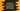# Introduction to JavaScript rest parameter## JavaScript rest parameter :

rest parameter was introduced in ES2015 or ES6. It is a different way to take arguments in a function. Using rest parameter, we can receive infinite amount of arguments to a function. It takes the arguments as an array, so array operations are possible on it.

## Syntax of rest parameter :

rest parameter like other parameters prefixed with three dots (…). We can have one rest parameter with or without any other arguments. If we put it with other arguments, it should always be at the end. The syntax of a function with two parameters and one rest parameter looks like as below :

``````function n(x,y,...params){

}``````

Here, x and y are normal parameters and params is a rest parameter.

### Example of rest parameter :

``````function findAverage(...args) {
let sum = 0;
args.forEach(e => sum += e);
return sum / args.length;
}

console.log(findAverage(1, 2, 3));
console.log(findAverage(1, 2, 3, 4, 5));``````

In this example, we are finding the average value of an array of numbers. findAverage method has one rest parameter and we are passing variable number of arguments to it. As I have explained to you before that it takes the arguments as an array, we can do array operations on it. In this example, we are using forEach to iterate through the array elements and finding out the sum of all.

It will print the below output:

``````2
3``````

### Rest parameter with other parameters :

We can use rest parameters with other normal parameters. But the only thing is that we need to put it at the end.

For example :

``````function printDetails(first,second,...args) {
console.log('first :'+first)
console.log('second :'+second)
console.log('length of other args :'+args.length)
}

printDetails(1, 2, 3);
printDetails(1, 2, 3, 4, 5);``````

Here, the first and the second parameters are normal parameters. The third parameter is a rest parameter. If you run this program, it will print the below output :

``````first :1
second :2
length of other args :1
first :1
second :2
length of other args :3``````